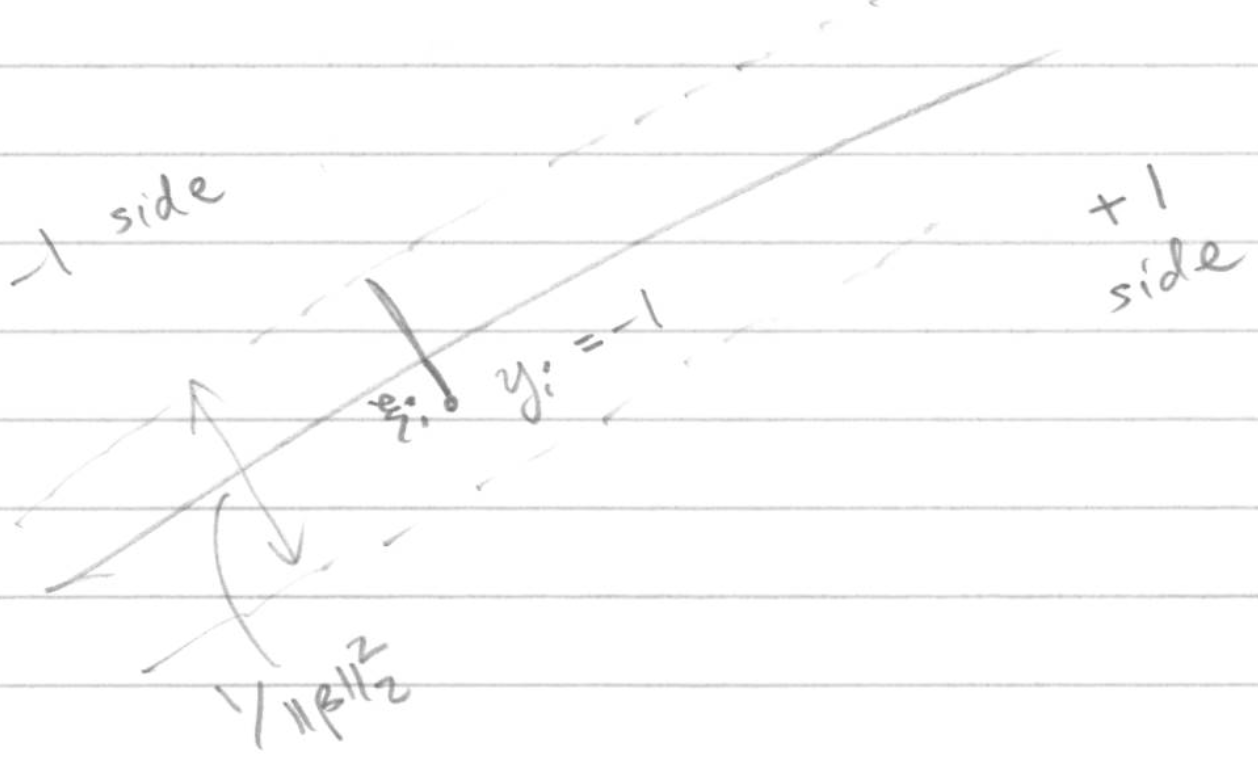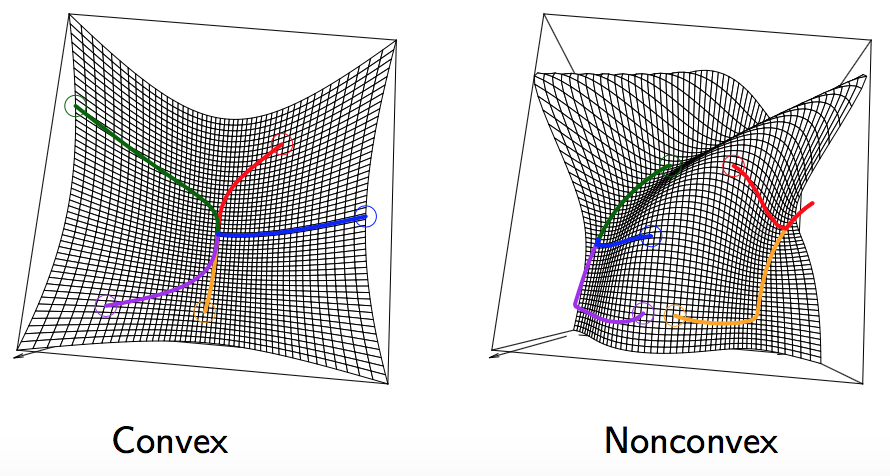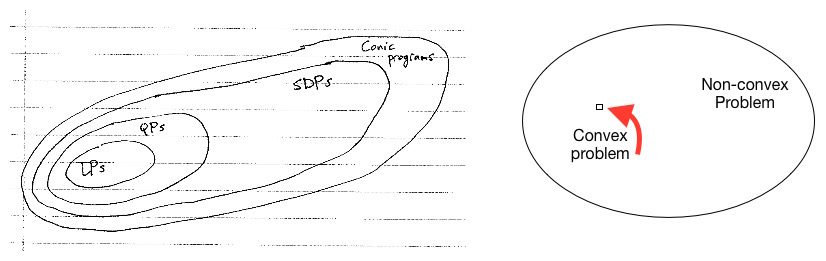# 凸优化－优化问题

Posted by Longfei Han on September 25, 2015

### 1. 凸优化问题

\begin{split} \min \limits_{x \in D} \quad & f(x) \
subject \, to \quad & g_i(x) \leq 0, \, i=1, \ldots, m \
& h_j(x)=0, \, j=1, \ldots, r \end{split}

• f被称为目标函数（objective or criterion function）；

• g被称为约束函数（inequality constraint function）；

• 满足$$g_i(x) \leq 0$$且$$h_j(x)=0$$的点称为可行解（feasible point）；

• 所有可行解中函数$$f(x)$$最小的点称为最优值（optimal value），记为$$f^{\ast}$$；

• 使得函数$$f(x)$$取得$$f^{\ast}$$的点$$x$$称为最优解（optimal point、solution或minimizer），当然，不是所有凸函数都存在最优解，即解为空，例如直线就不存在；

• 如果点$$x$$满足$$f(x) \leq f^{\ast} + \epsilon$$，则称点$$x$$为$$\epsilon-suboptimal$$解。

### 2. 凸优化解的集合

\begin{aligned} X_{opt} = \quad & argmin \, f(x) \
& subject \, to \, g_i(x) \leq 0, \, i=1, \ldots, m\
& Ax=b \end{aligned}

1. 凸优化解的集合有一个关键性质就是凸优化解的集合必为凸集。因为，如果凸优化问题不存在最优解，那么解空间为空集，为凸集；如果凸优化问题仅存在一个解，那必定为多维上的一点，而空间上的一点属于凸集；如果存在两个解，那么必定存在一条线段或者一个平面的解的集合，而线段或者平面都是凸集。接下来，就证明为什么存在两个解，就必然会存在多个解：

• $$g_i(\theta x + (1 - \theta)y) \leq \theta g_i(x)+(1-\theta)g_i(y) \leq 0$$;

• $$A(\theta x + (1 - \theta)y)=\theta Ax+(1-\theta)Ay=b$$;

• $$f(\theta x + (1 - \theta)y) \leq \theta f(x) + (1-\theta)f(y) = \theta f_{\ast} + (1-\theta)f_{\ast}=f_{\ast}$$。

1. 凸优化解的集合还有另外一个性质就是：如果优化函数为严格凸函数，那么它的最优解必是唯一的，即解空间$$X_{opt}$$只包含一个元素，如二次优化问题。

### 3. 凸优化问题实例：LASSO

\begin{split} \min \limits_{\beta \in \mathbb{R}^p} \quad & \parallel y-X\beta \parallel_2^2 \
subject \, to \quad & \parallel \beta \parallel_1 \leq s \end{split}

LASSO是Tibshirani（对就是Tibshirani）在1996年JRSSB上的一篇文章上《Regression shrinkage and selection via lasso》提出的。所谓lasso，其全称是least absolute shrinkage and selection operator，其含义是在限制了$$\sum \parallel \beta \parallel_1 \leq s$$的情况下，求使得残差平和达到最小的参数的估值。Tibshirani指出，对于回归算法，当$$s$$足够小的时候，会使得某些回归系数的估值是0，可以起到变量选择的作用，是逐步回归的一种表现。

\begin{split} \min \limits_{\beta, \beta_0, \xi} \quad & \frac{1}{2}\parallel \beta \parallel_2^2 + C \sum_i^n \xi_i \
subject \, to \quad & \xi_i \geq 0, \, i=1, \ldots, n\
& y_i(x_i^T \beta+\beta_0) \geq 1-\xi_i, \, i=1,\ldots,n \end{split}### 4. 局部最小值就是全局最小值• $$y \in D$$，因为$$x \in D$$，同时$$z \in D$$，二者线性组合也必然存在于D；

• $$g_i(y)=tg_i(z)+(1-t)g_i(x) \leq 0$$，因为$$g_i(z)，g_i(x) \leq 0$$；

• $$h_i(j)=a_j^T(tz+(1-t)x)+b_j=a_j^T(tz+(1-t)x)+tb_j+(1-t)b_j=0$$。

$f(y) \leq tf(z) + (1-t)f(x)$

### 5. 凸优化问题的一些性质和Trick

• First-order optimality condition：对于凸优化问题$$min \, f(x), \, subject \, to \, x \in C$$，如果函数$$f$$可微，那么当且仅当满足下式时，可行解（feasible point）$$x$$为最优解。
$\nabla f(x)^T (y-x) \geq 0 \quad \forall y \in C$
• Partial optimization：如果$$x=(x_1,x_2) \in \mathbb{R}^{n_1+n_2}$$，那么优化问题

\begin{split} \min \limits_{x_1,x_2} \quad & f(x_1,x_2) \
s.t. \quad & g_1(x) \leq 0, \
& g_2(x_2) \leq 0 \end{split}

等价于：

\begin{split} \min \limits_{x_1} \quad & \tilde{f}(x_1) \\ s.t. \quad & g_1(x_1) \leq 0 \end{split}

其中$$\tilde{f}(x_1)=min\{ f(x_1,x_2):g_2(x_2) \leq 0 \}$$；

SVM采用的hinge loss就是利用的partial optimization的思想。如果我们把SVM优化问题的目标函数记为：

\begin{split} \min \limits_{\beta,\beta_0,\xi} \quad & \frac{1}{2}\parallel \beta \parallel_2^2 + C \sum_i^n \xi_i \
subject \, to \quad & \xi_i \geq 0, \, y_i(x_i^T \beta + \beta_0) \geq 1-\xi_i \end{split}

那么我们可以将约束改写为$$\xi_i \geq max\{0, \, 1-y_i(x_i^T \beta + \beta_0) \}$$，SVM在优化过程中选用的hinge form就是将约束中的大于等于改写为等于，即:

$\xi_i = max \{ 0, \, 1-y_i(x_i^T \beta + \beta_0) \}$

因此，优化目标函数就变为：

$\min \limits_{\beta,\beta_0} \quad \frac{1}{2}\parallel \beta \parallel_2^2+C \sum_{i=1}^n[1-y_i(x_i^T \beta + \beta_0)]_+$

上式就是SVM求解目标函数的最终形式，可称为hinge form of SVMs

• Transformations of variables：如果函数$$h$$为单调递增函数，那么凸优化问题等价于：
$min\, f(x), \, subject\, to\, x \in C \Longleftrightarrow min \, h(f(x)), \, subject\, to\, x \in C$

优化方法中的最大似然估计MLE就采用log函数对目标函数进行变换，就是采用的这个思想。

• Introducing slack variables：凸优化可以通过引入松弛因子（slack variables）来消除约束（constraints）中的不等式，我们可以把凸优化问题转换为：

\begin{split} min \quad & f(x) \
subject\, to \quad & s_i \geq 0, i=1,\ldots,m\
& g_i(x)+s_i=0, i=1,\ldots,m\
& Ax=b \end{split}

SVM算法都引入slack variables来允许训练误差的出现，防止模型过拟合。

### 5. 凸优化问题分类

• linear programs：线性规划；
• Semidefinite programs：半正定规划；
• Cone programs：锥规划。

Ryan教授给了一个非常形象的例子来解释凸优化问题在优化问题领域的位置，以及以上几种优化问题间的关联关系，如下图：\begin{split} \min \limits_{x} \quad & c^Tx \
subject \, to \quad & Dx \leq d \
& Ax =b \end{split}

\begin{split} \min \limits_{x} \quad & C^Tx+\frac{1}{2}x^TQx \
subject \, to \quad & Dx \leq d \
&Ax=b \end{split}

\begin{split} \min \limits_{x} \quad & c^Tx \
subject \, tp \quad & x_1F_1+\ldots +x_nF_n \succeq F_0 \
&Ax=b \end{split}